# DevExpress Charts for Xamarin.Forms

The DevExpress Charts for Xamarin.Forms suite helps you transform data to its most appropriate, concise, and readable visual representation.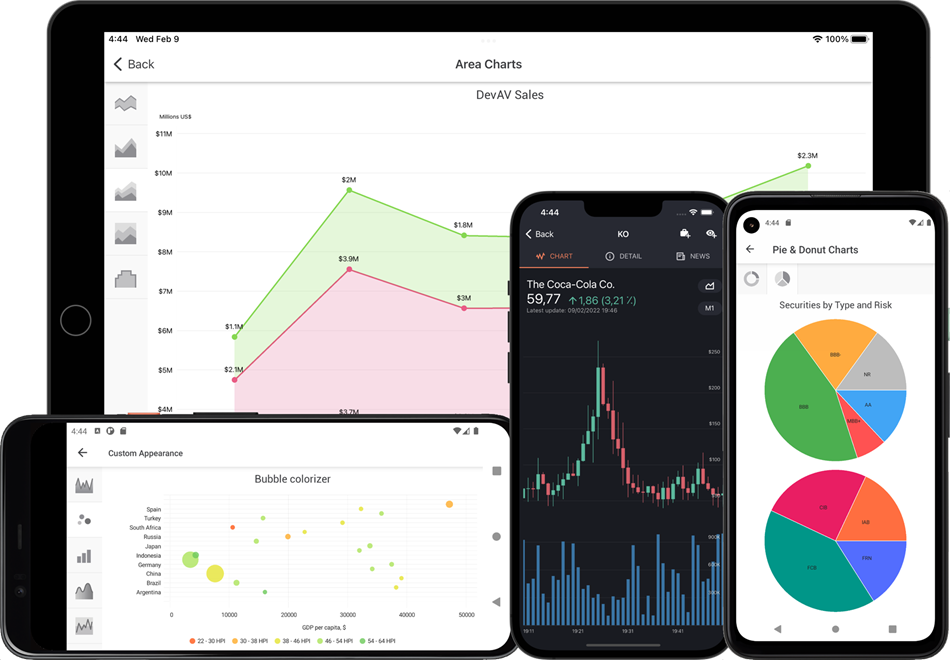Download and install the `DevExpress.XamarinForms.Charts` package from the DevExpress NuGet Gallery to obtain our Charts for Xamarin.Forms suite. The package contains the following components:

• ChartView—allows you to display data as bars, lines, areas, financial open-high-low-close views, and much more.

• PieChartView—allows you to visualize your data as pies and doughnuts.

To specify how data should be visualized, use series. This topic lists all supported series types.

## Point Series

You can display data as points in a Cartesian coordinate system. The image below illustrates two sample point series.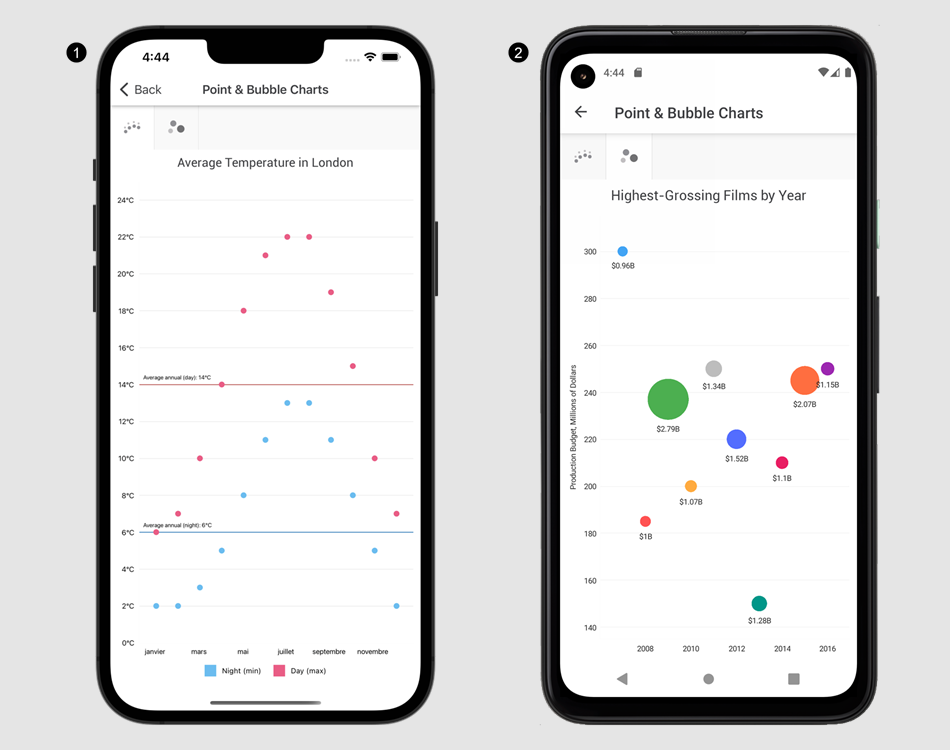1. Point Series
2. Bubble Series

The table below describes these two point series types.

Series Description
PointSeries Displays data as a collection of points.
BubbleSeries Displays three-dimensional data as a collection of bubbles, so that each bubble position visualizes the first two dimensions and the bubble size is the third dimension.

## Line Series

A chart can connect points in a Cartesian coordinate system to display data as lines. The image below illustrates two sample line series.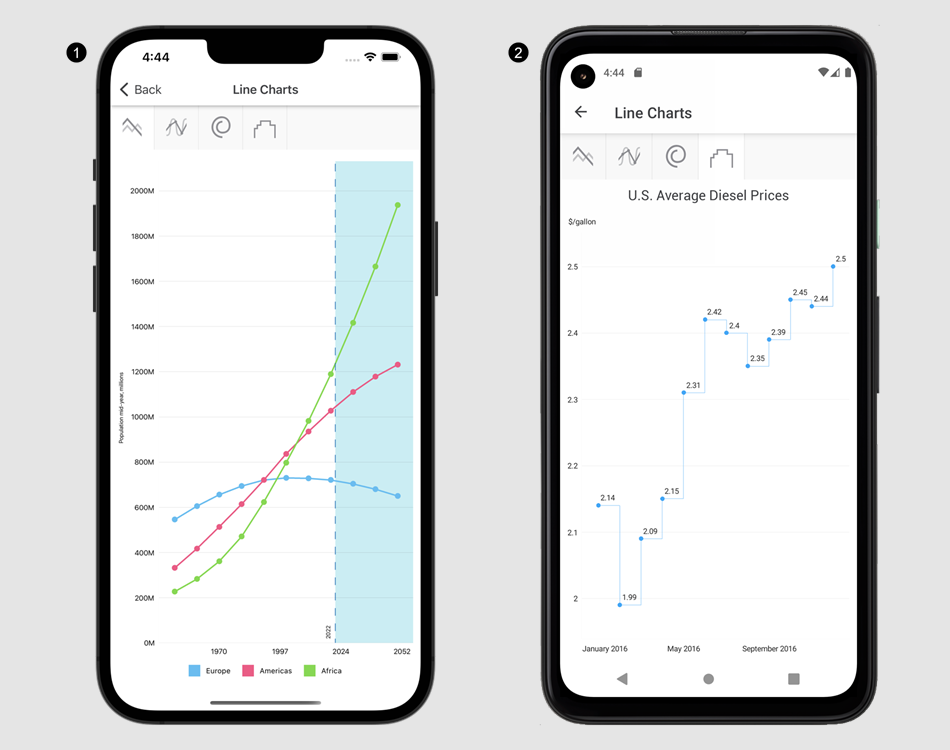1. Line Series
2. Step Line Series

The table below describes the supported line series types.

Series Description
LineSeries Displays data as points connected by a line.
SplineSeries Displays data as points connected by a curved line.
StepLineSeries Displays data as points connected by horizontal and vertical lines.
ScatterLineSeries Displays data as a collection of points connected by a line, in the order they were indexed (opposite to LineSeries that automatically sorts all series points within the collection by their arguments).

## Area Series

You can display data as filled areas on a chart. The image below illustrates two sample area series.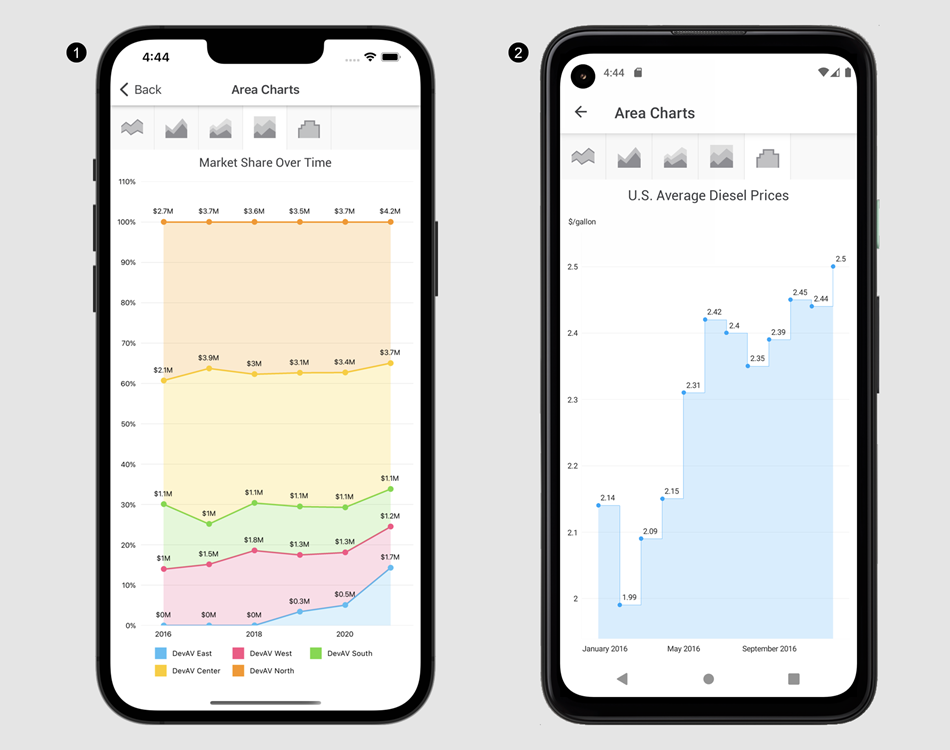1. Full Stacked Area Series
2. Step Area Series

The table below describes the supported area series types.

Series Description
AreaSeries Displays data as filled areas on a chart, with each data point as a peak or hollow in this filled area.
RangeAreaSeries Displays data as a filled area between two value sets.
StackedAreaSeries Displays data as areas on a chart, so that each data point value is aggregated with values of the underlying data points.
FullStackedAreaSeries Displays data as areas on a chart, so that each data point value is stacked with values of all the other corresponding data points and the total area is always the full area of the chart diagram.
StepAreaSeries Displays data as filled areas on a chart, with data points connected by horizontal and vertical lines.

## Bar Series

This series type displays data values as individual bars. The image below illustrates two sample bar series.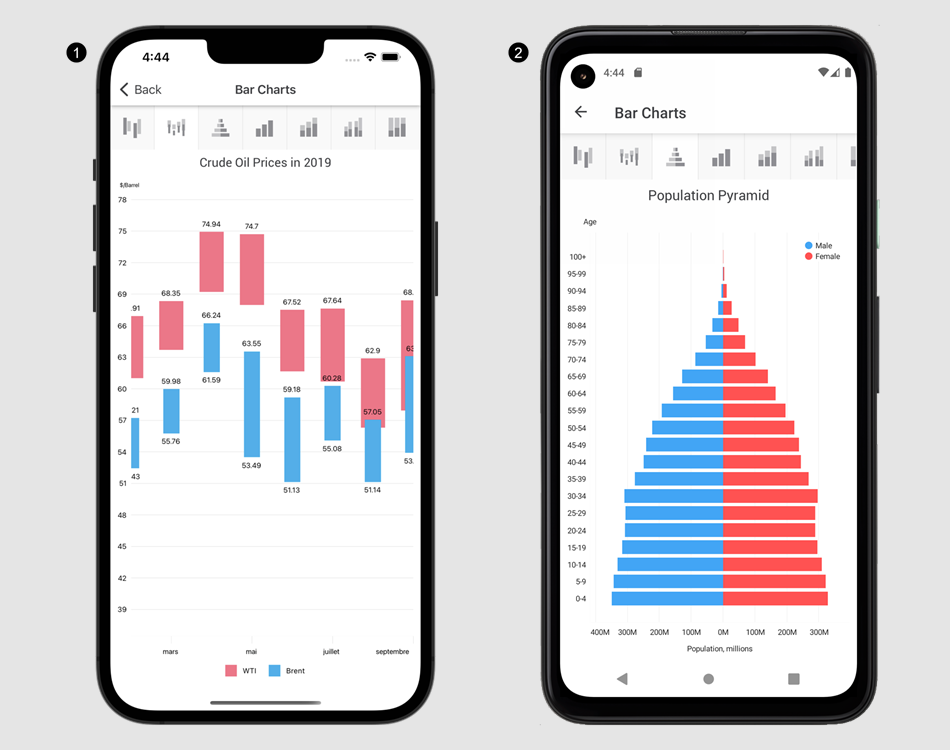1. Range Bar Series
2. Stacked Bar Series

The table below describes the supported bar series types.

Series Description
BarSeries Displays data as individual bars grouped by arguments, and each bar height is determined by the data value.
StackedBarSeries Displays data as bars stacked by arguments, so that each stacked bar height is determined by the total of all the series values for the argument.
FullStackedBarSeries Displays data as bars stacked by arguments, so that each stacked bar height is always the full height of the chart diagram and series values are displayed as percentages of a bar.
SideBySideStackedBarSeries Displays data as bars stacked according to the StackedGroup property value and grouped by arguments. The height of each stacked bar is determined by the total of series values the bar contains.
SideBySideFullStackedBarSeries Displays data as bars stacked according to the StackedGroup property value and grouped by arguments. The height of each bar is always the full height of the chart diagram and series values are displayed as percentages of a bar.
RangeBarSeries Displays data as bars that show ranges between two data values for argument values. Bars with the same argument from different series may overlap.
SideBySideRangeBarSeries Displays data as bars grouped by arguments. Each bar shows the range between two data values for the argument value.

## Pie Series

The pie and donut series illustrate the numerical proportion of data values.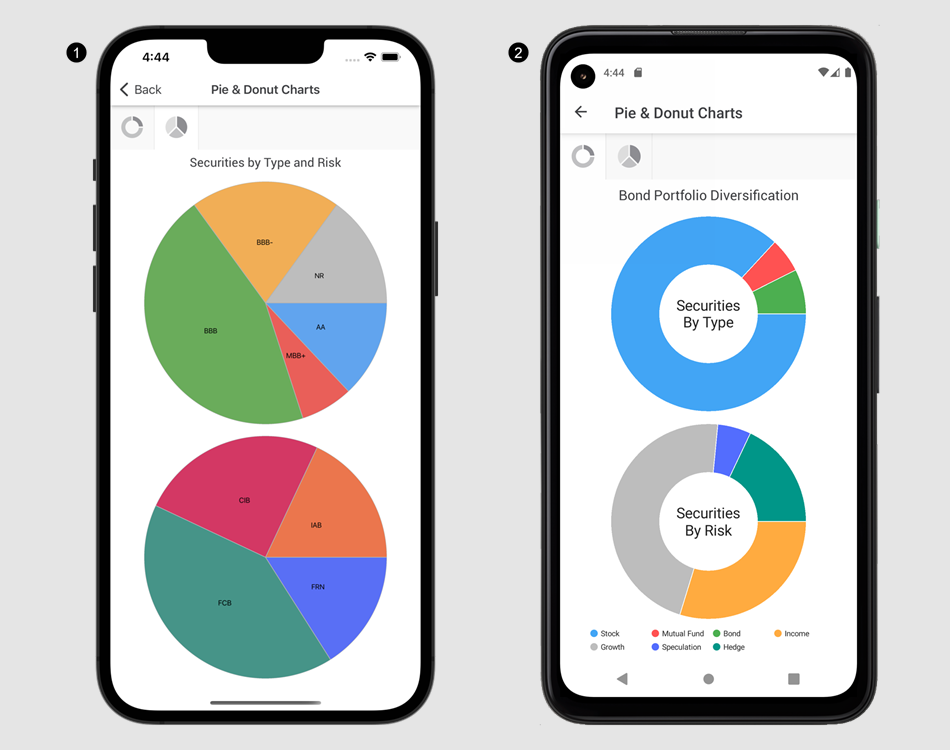1. Pie Series
2. Donut Series

The table below describes these two pie series types.

Series Description
PieSeries Displays data as circular graphic divided into slices to illustrate numerical proportion.
DonutSeries Displays data as a pie chart with a hole in center.

Note

Use the PieChartView to display a PieSeries or DonutSeries.

## Financial Series

The financial series allows you to display Open-High-Low-Close prices and calculate volatility, average, deviation, and many other indicators.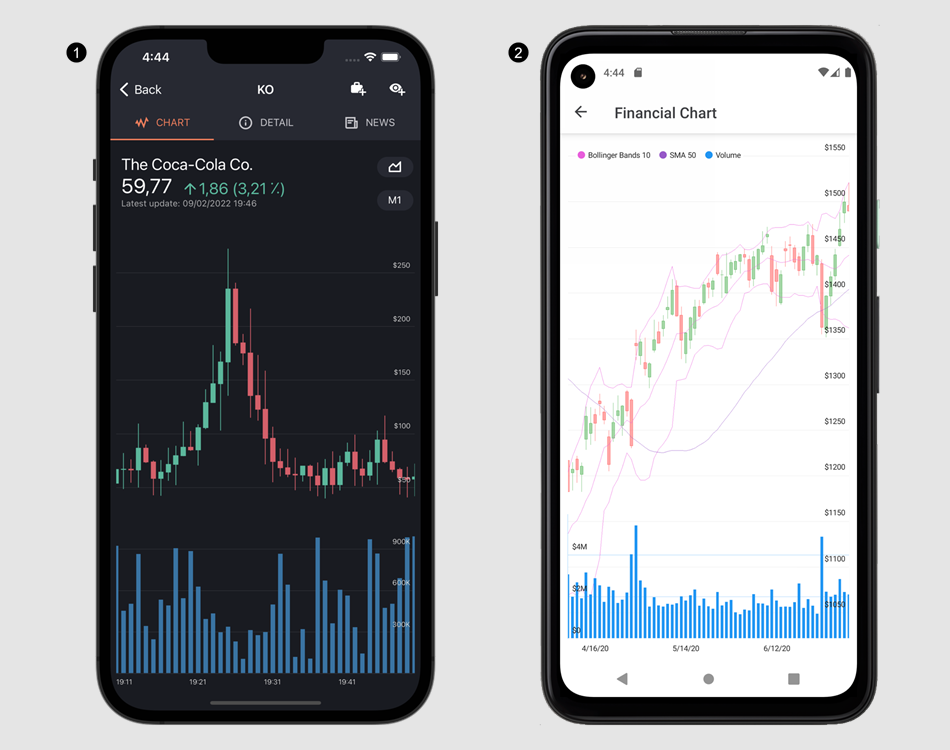1. Candle Stick Series, Bar Series
2. Candle Stick Series, Bollinger Bands Indicator, Moving Average Indicator, Bar Series

The table below describes the supported financial series and indicator types.

Series/Indicator Description
StockSeries Displays an Open-High-Low-Close typical financial series so that each data point consists of a vertical line indicating the price range over one unit of time, and the left and right tick marks showing the opening and closing prices for that time period, respectively.
CandleStickSeries Displays an Open-High-Low-Close typical financial series so that each data point consists of a vertical line indicating the price range over one unit of time and a rectangle showing the opening and closing prices for that time period.
BollingerBandsIndicator A Bollinger Bands indicator.
MovingAverageIndicator A simple moving average (SMA) indicator.
ChaikinsVolatilityIndicator A Chaikin’s volatility (CHV) indicator.
EnvelopeIndicator A simple moving average envelope.
ExponentialMovingAverageIndicator An exponential moving average (EMA) indicator.
WeightedMovingAverageIndicator A weighted moving average (WMA) indicator.
TriangularMovingAverageIndicator A triangular moving average (TMA) indicator.
RateOfChangeIndicator A rate of change (ROC) indicator.
RegressionLineIndicator A regression line indicator.
WeightedCloseIndicator A weighted close indicator.
WilliamsRIndicator A Williams %R indicator.
AverageTrueRangeIndicator An average true range (ATR) indicator.
CommodityChannelIndexIndicator A commodity channel index (CCI) indicator.
MassIndexIndicator A mass index indicator.
MedianPriceIndicator A median price indicator.
MovingAverageConvergenceDivergenceIndicator A moving average convergence/divergence (MACD) indicator.
TypicalPriceIndicator A typical price indicator.
RelativeStrengthIndexIndicator A relative strength index (RSI) indicator.
StandardDeviationIndicator A standard deviation indicator.